#### You may also like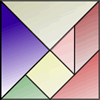How can the same pieces of the tangram make this bowl before and after it was chipped? Use the interactivity to try and work out what is going on!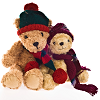### Teddy Town

There are nine teddies in Teddy Town - three red, three blue and three yellow. There are also nine houses, three of each colour. Can you put them on the map of Teddy Town according to the rules?### Four Triangles Puzzle

Cut four triangles from a square as shown in the picture. How many different shapes can you make by fitting the four triangles back together?

# Rod Ratios

## Rod Ratios

You may like to use our Cuisenaire rod environment for the problem below. Here is the environment (just scroll down for the problem itself):

Here are three pairs of rods. The ratio of all the pairs is 3 : 2.What is the ratio of the pair below?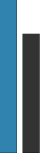Using only single rods what pair can you find with the same ratio as the pair below?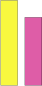Using only pairs of single rods what pairs can you find with the same ratio as those below?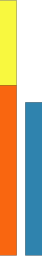Using only pairs of single rods what pairs can you find with the ratio 9 : 6?

### Why do this problem?

Cuisenaire rods are a fantastic resource for investigating ratio.

### Possible approach

The way the problem is written assumes knowledge of the concept of ratio, but Cuisenaire rods could be used to introduce the ideas initially. Children will find it useful to have some "real" rods to use while working on the problem, and the interactivity can be a good medium in which to engage the whole class in initial discussion, perhaps on an interactive whiteboard or using a projector. White rods (which are unit length) can be used to demonstrate what exactly a ratio of 3:2 means and there is a lot to be talked about in terms of why 3:2 is the same as 6:4, 9:6 and so on.